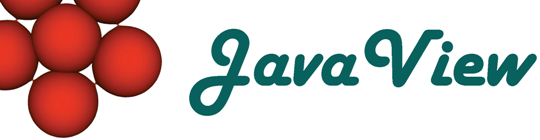Overview  Gallery  Introduction  Objects  Packages  Packages 3D  Basic Options  3D Options  Advanced

## 4. Working with Graphics3D Packages

### Standard

Mathematica provides built-in commands to render function graphs, parametrized surfaces and curves in 3D.

#### Function Graph

 Graph of a scalar function on a rectangular domain. In:= fg = Plot3D[y/6 Sin[x], {x, -Pi, Pi}, {y, 0., 6}] In:= JavaView[fg] Compare with Mathematica

#### Parametrized Surfaces

 Sphere in standard parametrization with meridians and parallels. In:= gp = ParametricPlot3D[{Cos[u] Cos[v], Sin[u] Cos[v], Sin[v]}, {u, 0, 2Pi}, {v, -Pi/2, Pi/2}, PlotPoints -> {40, 10}]; In:= JavaView[gp] Compare with Mathematica

#### 3D Curves

 A closed curve in space. In:= cv = ParametricPlot3D[{Cos[5t], Sin[3t], Sin[t]}, {t, 0, 2 Pi}]; In:= JavaView[cv] Compare with Mathematica

### Graphics`ContourPlot3D`

Compute implicit surfaces as zero set of a function or of a scalar values given at vertices of a ZxZxZ grid.

 Zero level of a function. In:= <
 Zero level of a regular scalar field in 3D. In:= < {{-1, 1}, {-1, 1}, {-1, 1}}, Lighting -> False, Contours -> {1.5, 3.}, Axes -> True, ContourStyle -> {{RGBColor[0, 1, 0]}, {RGBColor[1, 0, 0]}}] In:= JavaView[gl] Compare with Mathematica

### Graphics`Graphics3D`

Package supplies different representations of 3D data like bar chart and shadow plots.

#### Bar Charts

Display scalar values on a uv-mesh as vertical columns whose height represent the value.

 This gives a bar chart made from a two-by-three array of bars with integer height. In:= < Automatic]; In:= JavaView[gb] Compare with Mathematica

#### ScatterPlot3D

ScatterPlot3D[] shows a set of 3D points and thereby extends ListPlot to 3D. Optionally, the points may be connected by a line.

 Here is a list of points in three dimensions. The scatter plot of points lies on a conical helix. In:= <
 Join the points of the same list with a thicker line. In:= < True, PlotStyle -> Thickness[0.01]] In:= JavaView[scl] Compare with Mathematica

ListSurfacePlot3D uses an array of points and generates the faces of a surface.

 Take an array of points in three dimensions. The array of points is used to generate vertices of a polygonal mesh. It creates a piece of a sphere. In:= <

Draw shadows of a surface at its bounding box. ShadowPlot3D and ListShadowPlot3D work exactly like the built­in Plot3D and ListPlot3D, except shadows are drawn.

 Plot a 2D shadow below a 3D surface. In:= <
 ShadowPosition determines whether shadow is above or below. In:= < 1] In:= JavaView[st] Compare with Mathematica
 Plot shadows at the bounding box of a 3D surface. In:= < None];In:= sa := Shadow[dbell, ZShadow -> False]; In:= JavaView[sa] Compare with Mathematica

### Graphics`ParametricPlot3D`

The command ParametricPlot3D[] in this package expands the built-in command by allowing du and dv increments to be specified.

 Determine number of lines through du and dv instead of PlotPoints. In:= <
 Only a collection of points is shown when you use PointParametricPlot3D. In:= <

### Graphics`PlotField3D`

Creates and renders vector fields in 3D.

 A 3D vortex vector field. In:= <
 The same vector field with VectorHeads. In:= < True] In:= JavaView[gv3h] Compare with Mathematica

### Graphics`Polyhedra`

This package contains a collection of Platonic solids and commands for their modification like Stellate[] and Truncate[].

 Load package Graphics`Polyhedra and display a dodecahedron in a JavaView display. In:= <
 Truncate the dodecahedron. In:= tg = Truncate[dode]; In:= JavaView[tg] Compare with Mathematica
 Stellate the remainder. In:= ts = Stellate[tg]; In:= JavaView[ts] Compare with Mathematica

### Graphics`Shapes`

A collection of primitive surfaces including sphere, torus, Mbius band etc. is provided by this package.

 Combine two shapes and position them in a scene. JavaView accepts a list of geometries. In:= << Graphics`Shapes` In:= g1 = TranslateShape[Graphics3D[Torus[]], {1., 0., 0.}];In:= g2 = RotateShape[Graphics3D[MoebiusStrip[1., 0.2, 30]], Pi/2., Pi/2., 0.]; In:= JavaView[{g1,g2}] Compare with Mathematica

### Graphics`SurfaceOfRevolution`

This package simplifies the task to generate a surface of revolution from a planar (or non-planar) curve by rotation around the default z-axis, or about a user specified rotation axis.

 The curve Sin[x] is rotated around the z-axis with an offset. In:= << Graphics`SurfaceOfRevolution`In:= g = SurfaceOfRevolution[{1.2 + Sin[x], x}, {x, 0, 2Pi}]; In:= JavaView[g] Compare with Mathematica

The Pseudosphere is obtained by rotating a tractix around the z-axis.

 Rotate the tractrix {f[x],g[x]} around the z-axis. In:= << Graphics`SurfaceOfRevolution`In:= gPsd = SurfaceOfRevolution[{ Exp[-Abs[x]], -Sign[x](Sqrt[1 - Exp[-2 Abs[x]]] - Log[(1 + Sqrt[1 - Exp[-2 Abs[x]]])/Exp[-Abs[x]]])}, {x, -2., 2.}] In:= JavaView[gPsd] Compare with Mathematica